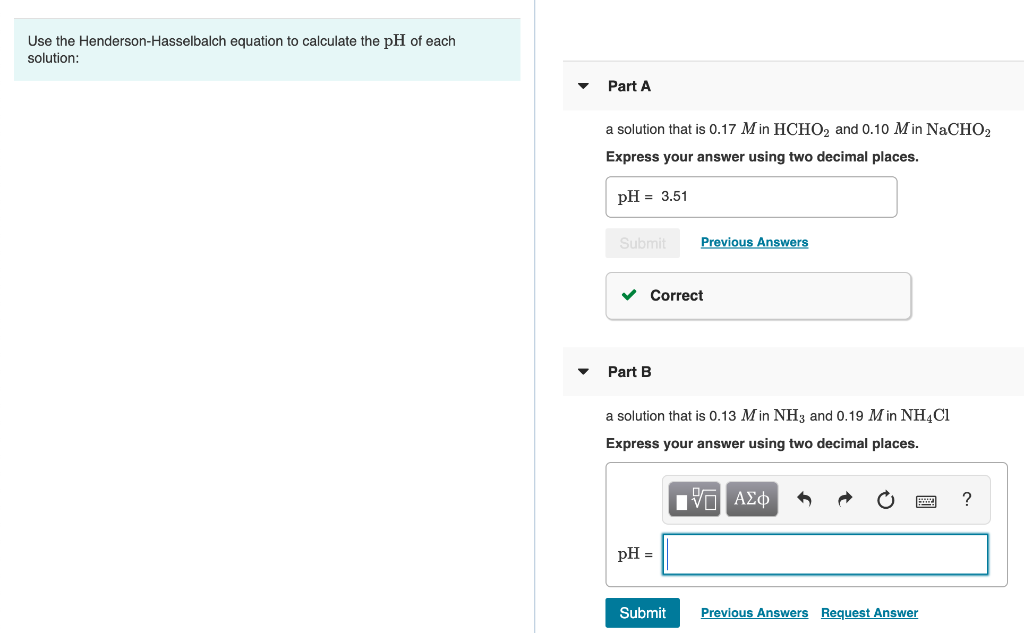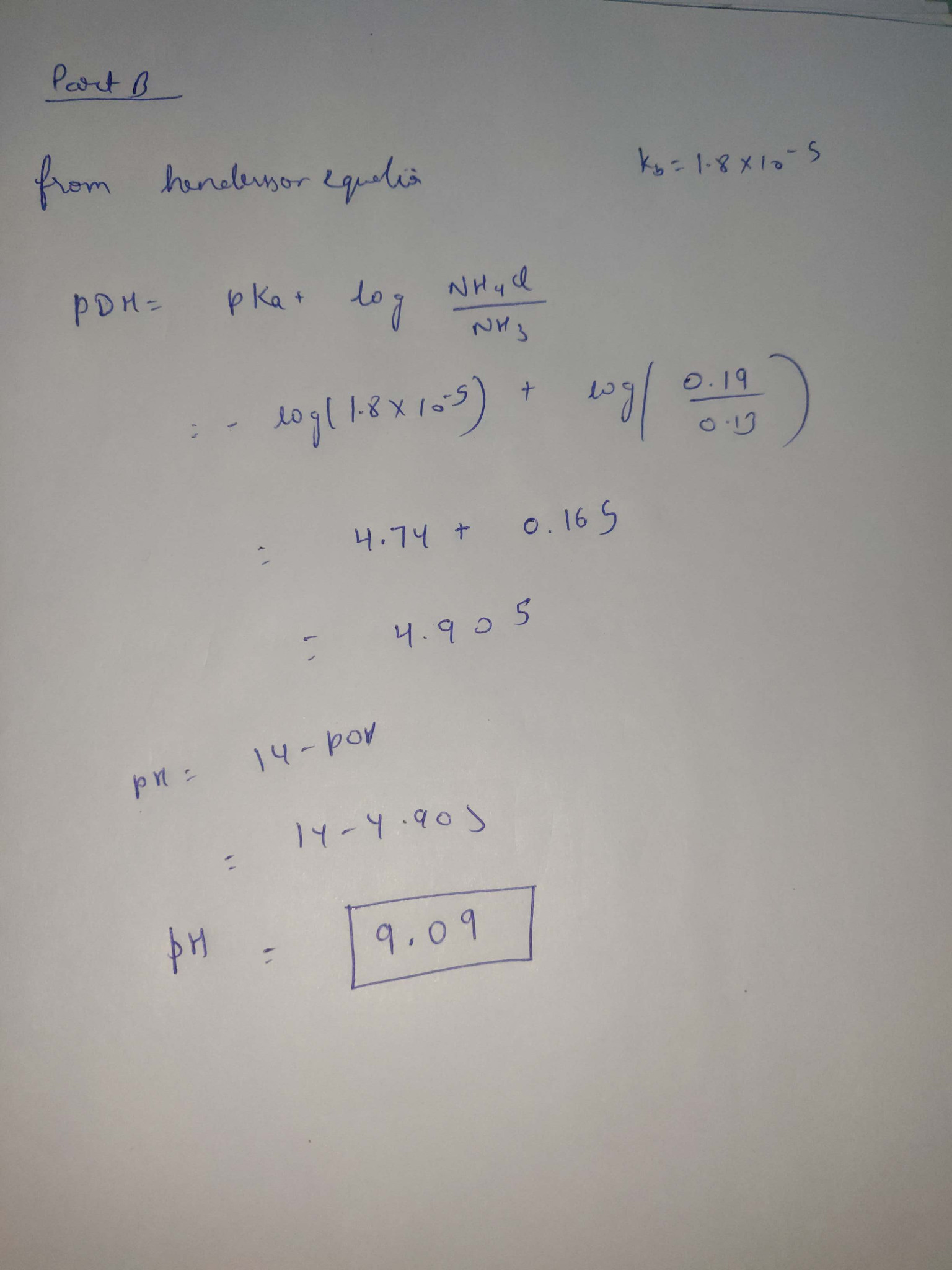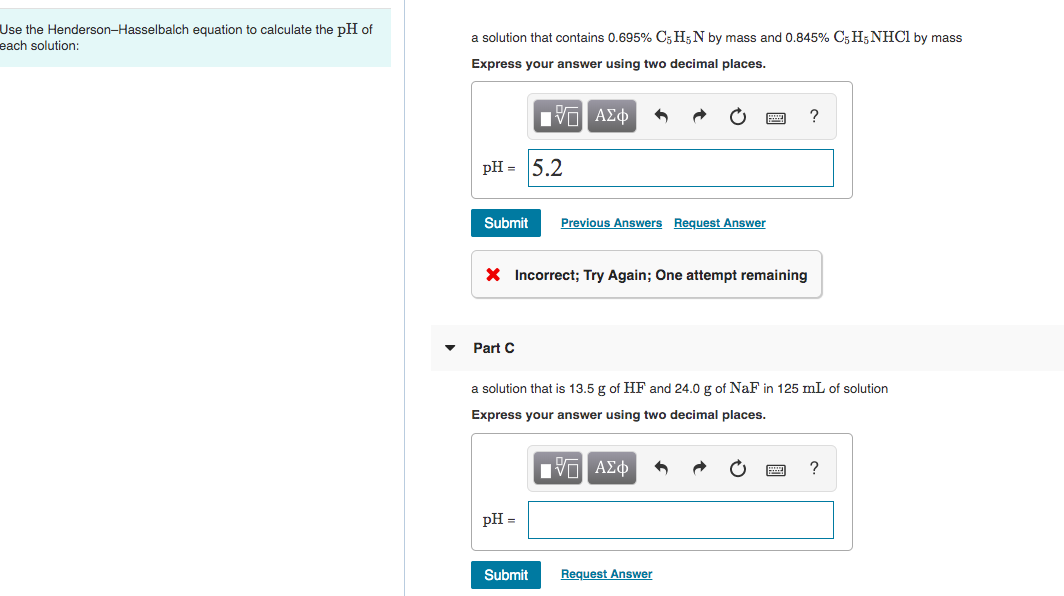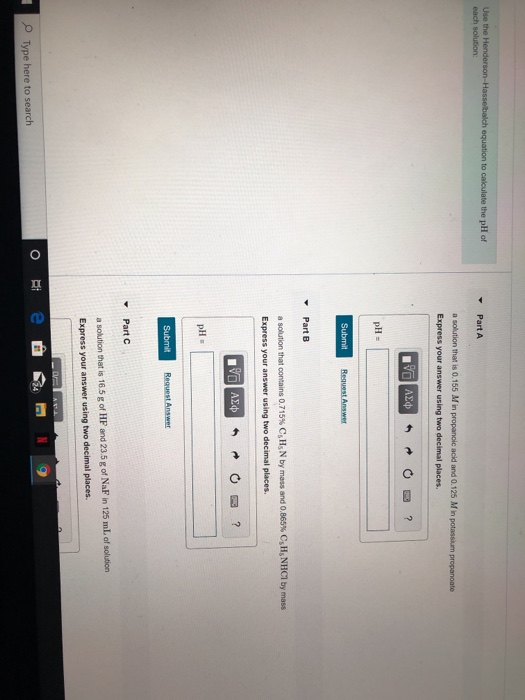# Use the Henderson-Hasselbalch equation to calculate the pH of each solution: Part A a solution that...Use the Henderson-Hasselbalch equation to calculate the pH of each solution: Part A a solution that is 0.17 Min HCHO2 and 0.10 Min NaCHO2 Express your answer using two decimal places. pH = 3.51 Previous Answers ✓ Correct Part B a solution that is 0.13 Min NH3 and 0.19 M in NH4Cl Express your answer using two decimal places. I AM A O 2 ? pH = | Submit Previous Answers Request Answer#### Earn Coin

Coins can be redeemed for fabulous gifts.

Similar Homework Help Questions
• ### Use the Henderson-Hasselbalch equation to calculate the pH of each solution: (a) a solution that is...

Use the Henderson-Hasselbalch equation to calculate the pH of each solution: (a) a solution that is 0.20 M in HCHO2 and 0.10 M in NaCHO2. (Ka=1.8×10−4) (b) a solution that is 0.14 M in NH3 and 0.19 M in NH4Cl. (Kb=1.76×10−5)

• ### Use the Henderson-Hasselbalch equation to calculate the pH of each solution: A. a solution that is...

Use the Henderson-Hasselbalch equation to calculate the pH of each solution: A. a solution that is 0.14 M in HCHO2 and 0.13 M in NaCHO2 B. a solution that is 0.15 M in NH3 and 0.18 M in NH4Cl

• ### Use the Henderson-Hasselbalch equation to calculate the pH of each solution: A. a solution that is...

Use the Henderson-Hasselbalch equation to calculate the pH of each solution: A. a solution that is 0.14 M in HCHO2 and 0.13 M in NaCHO2 B. a solution that is 0.15 MM in NH3NH3 and 0.18 MM in NH4Cl

• ### Use the Henderson-Hasselbalch equation to calculate the pH of each solution: a solution that contains 0.695%...Use the Henderson-Hasselbalch equation to calculate the pH of each solution: a solution that contains 0.695% C5H5N by mass and 0.845% C5H5NHCI by mass Express your answer using two decimal places. Hν ΑΣφ ? рH- 15.2 Previous Answers Request Answer Submit Incorrect; Try Again; One attempt remaining Part C a solution that is 13.5 g of HF and 24.0 g of NaF in 125 mL of solution Express your answer using two decimal places. ΑΣφ ? pH Request Answer Submit

• ### Use the Henderson-Hasselbalch equation to calculate the pHpH of each solution: Part A a solution that...

Use the Henderson-Hasselbalch equation to calculate the pHpH of each solution: Part A a solution that is 0.19 MM in HCHO2HCHO2 and 0.10 MM in NaCHO2

• ### calculate pH using Henderson-Hasselbalch equation

Use the Henderson-Hasselbalch equation to calculate the pH of each of the following solutions.a. a solution that is 0.17 in and 0.11 in b. a solution that is 0.13 in and 0.19 in

• ### I need a, b and c please. Thanks! Use the Henderson-Hasselbalch equation to calculate the pH...I need a, b and c please. Thanks! Use the Henderson-Hasselbalch equation to calculate the pH of each solution: Part A a solution that is 0.155 M in propanoic acid and 0.125 Min potassium propanoate Express your answer using two decimal places. VO ALTRO? pH = Submit Request Answer Part B a solution that contains 0.715% C, H N by mass and 0.865% CsHNHCl by mass Express your answer using two decimal places. V AED RO? pH- Submit Request Answer...

• ### Use the Henderson-Hasselbalch equation to calculate the pH of each solution: A solution that is 0.14...

Use the Henderson-Hasselbalch equation to calculate the pH of each solution: A solution that is 0.14 M in NH3 and 0.20 M in NH4Cl. (Kb= 1.76x10^-5)

• ### Henderson-Hasselbalch equation and pH , mass HCl could buffer neutralize

1. Use the Henderson-Hasselbalch equation to calculate the pH of a solution that is 0.195 M in C2H5NH2 and 0.155 M in C2H5NH3Br.Express your answer using two decimal places.pH = ? (I entered 10.79 and there was a message that Not quite. Check through your calculations; you may have made a rounding error or used the wrong number ofsignificant figures.)2. Use the Henderson-Hasselbalch equation to calculate the pH of a solution that is 12.5 g of HC2H3O2 and 13.5 g...

• ### 1)Use the Henderson–Hasselbalch equation to calculate the pH of: a solution that is 13.5 g of...

1)Use the Henderson–Hasselbalch equation to calculate the pH of: a solution that is 13.5 g of HF and 25.0 g of NaF in 125 mL of solution Express your answer using two decimal places. 2) A volume of 20.0 mL of a 0.380 M HNO3 solution is titrated with 0.850 M KOH. Calculate the volume of KOH required to reach the equivalence point. Express your answer to three significant figures and include the appropriate units.